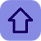## INTERVIEW QUESTIONS IN "C PROGRAMMING "LANGUAGE

Posted by Tushar Bedekar

### CHAPTER 1: Variables & Control Flow

1. What is the difference between declaring a variable and defining a variable?
2. What is a static variable?3. What is a register variable?
4. Where is an auto variable stored?
5. What is scope & storage allocation of extern and global variables?
6. What is scope & storage allocation of register, static and local variables?
7. What are storage memory, default value, scope and life of Automatic and Register storage class?
8. What are storage memory, default value, scope and life of Static and External storage class?
9. What is the difference between 'break' and 'continue' statements?
10. What is the difference between 'for' and 'while' loops?

### CHAPTER 2: Operators, Constants & Structures

1. Which bitwise operator is suitable for checking whether a particular bit is ON or OFF?
2. Which bitwise operator is suitable for turning OFF a particular bit in a number?
3. What is equivalent of multiplying an unsigned int by 2: left shift of number by 1 or right shift of number by
1?
4. What is an Enumeration Constant?
5. What is a structure?
6. What are the differences between a structure and a union?
7. What are the advantages of unions?
8. How can typedef be to define a type of structure?
9. Write a program that returns 3 numbers from a function using a structure.
10. In code snippet below:
struct Date {
int yr;
int day;
int month;
} date1,date2;
date1.yr = 2004;
date1.day = 4;
date1.month = 12;
Write a function that assigns values to date2. Arguments to the function must be pointers to the
structure, Date and integer variables date, month, year.

### CHAPTER 3: Functions

1. What is the purpose of main() function?
2. Explain command line arguments of main function?
3. What are header files? Are functions declared or defined in header files ?
4. What are the differences between formal arguments and actual arguments of a function?
5. What is pass by value in functions?
6. What is pass by reference in functions?
7. What are the differences between getchar() and scanf() functions for reading strings?
8. Out of the functions fgets() and gets(), which one is safer to use and why?
9. What is the difference between the functions strdup() and strcpy()?

solution:-coming-soon

### CHAPTER 4: Pointers

1. What is a pointer in C?
2. What are the advantages of using pointers?
3. What are the differences between malloc() and calloc()?
4. How to use realloc() to dynamically increase size of an already allocated array?
5. What is the equivalent pointer expression for referring an element a[i][j][k][l], in a four dimensional array?
6. Declare an array of three function pointers where each function receives two integers and returns float.
7. Explain the variable assignment in the declaration
int *(*p)(char *, char *);
8. What is the value of
sizeof(a) /sizeof(char *)
in a code snippet:
char *a={"sridhar","raghava","shashi","srikanth"};
9. (i) What are the differences between the C statements below:
char *str = "Hello";
char arr[] = "Hello";
(ii) Whether following statements get complied or not? Explain each statement.
arr++;
*(arr + 1) = 's';
printf("%s",arr);

solution:-coming-soon

### CHAPTER 5: Programs

1. Write a program to find factorial of the given number.
2. Write a program to check whether the given number is even or odd.
3. Write a program to swap two numbers using a temporary variable.
4. Write a program to swap two numbers without using a temporary variable.
5. Write a program to swap two numbers using bitwise operators.
6. Write a program to find the greatest of three numbers.
7. Write a program to find the greatest among ten numbers.
8. Write a program to check whether the given number is a prime.
9. Write a program to check whether the given number is a palindromic number.
10.Write a program to check whether the given string is a palindrome.
11.Write a program to generate the Fibonacci series.
12.Write a program to print "Hello World" without using semicolon anywhere in the code.
13.Write a program to print a semicolon without using a semicolon anywhere in the code.
14.Write a program to compare two strings without using strcmp() function.
15.Write a program to concatenate two strings without using strcat() function.
16.Write a program to delete a specified line from a text file.
17.Write a program to replace a specified line in a text file.
18.Write a program to find the number of lines in a text file.
19.Write a C program which asks the user for a number between 1 to 9 and shows the number. If the user inputs a number out of the specified range, the program should show an error and prompt the user for a valid input.
20.Write a program to display the multiplication table of a given number.

solution:-coming-soon

## Try If You Can

Posted by Tushar Bedekar

• The responses May be Generated either using GCC or Turbo C compiler.It does`t matter but the correctness of responses is required.
• Online Compiler HERE

## C Program to Print 1-10 numbers without using Conditional Loops

Posted by Tushar Bedekar
Print 1-10 numbers without using Conditional Loop i.e without using
• for Loop
• while Loop
• do-while Loop

#include<stdio.h>

void printNumber(int value) {
int i;
printf("%d\n", value);          //The Following programme is written using the concept of recursion
i = value + 1;

if (i > 10)
return;
printNumber(i);
}

void main() {
printNumber(1);
}